Courses

# Moving Load & Its Effects on Structural Members - 1 Civil Engineering (CE) Notes | EduRev

## Civil Engineering (CE) : Moving Load & Its Effects on Structural Members - 1 Civil Engineering (CE) Notes | EduRev

The document Moving Load & Its Effects on Structural Members - 1 Civil Engineering (CE) Notes | EduRev is a part of the Civil Engineering (CE) Course Structural Analysis.
All you need of Civil Engineering (CE) at this link: Civil Engineering (CE)

Instructional Objectives:

The objectives of this lesson are as follows:

• Understand the moving load effect in simpler term
• Study various definitions of influence line
• Introduce to simple procedures for construction of influence lines

Introduction

In earlier lessons, you were introduced to statically determinate and statically indeterminate structural analysis under non-moving load (dead load or fixed loads). In this lecture, you will be introduced to determination of maximum internal actions at cross-sections of members of statically determinate structured under the effects of moving loads (live loads).

Common sense tells us that when a load moves over a structure, the deflected shape of the structural will vary. In the process, we can arrive at simple conclusion that due to moving load position on the structure, reactions value at the support also will vary.

From the designer’s point of view, it is essential to have safe structure, which doesn’t exceed the limits of deformations and also the limits of load carrying capacity of the structure.

Definitions of influence line

In the literature, researchers have defined influence line in many ways. Some of the definitions of influence line are given below.

• An influence line is a diagram whose ordinates, which are plotted as a function of distance along the span, give the value of an internal force, a reaction, or a displacement at a particular point in a structure as a unit load move across the structure.
• An influence line is a curve the ordinate to which at any point equals the value of some particular function due to unit load acting at that point.
• An influence line represents the variation of either the reaction, shear, moment, or deflection at a specific point in a member as a unit concentrated force moves over the member.

Construction of Influence Lines

In this section, we will discuss about the construction of influence lines. Using any one of the two approaches (Figure 37.1), one can construct the influence line at a specific point P in a member for any parameter (Reaction, Shear or Moment). In the present approaches it is assumed that the moving load is having dimensionless magnitude of unity. Classification of the approaches for construction of influence lines is given in Figure 37.1.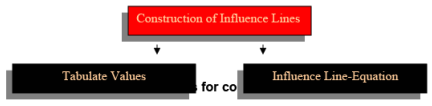Tabulate Values

Apply a unit load at different locations along the member, say at x. And these locations, apply statics to compute the value of parameter (reaction, shear, or moment) at the specified point. The best way to use this approach is to prepare a table, listing unit load at x versus the corresponding value of the parameter calculated at the specific point (i.e. Reaction R, Shear V or moment M) and plot the tabulated values so that influence line segments can be constructed.

Sign Conventions

Sign convention followed for shear and moment is given below.

 Parameter Sign for influence line Reaction R Positive at the point when it acts upward on the beam. Shear V Positive for the following case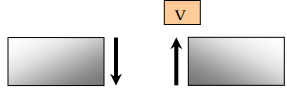Moment M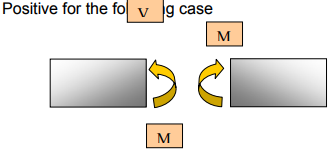Influence Line Equations

Influence line can be constructed by deriving a general mathematical equation to compute parameters (e.g. reaction, shear or moment) at a specific point under the effect of moving load at a variable position x.

The above discussed both approaches are demonstrated with the help of simple numerical examples in the following paragraphs.

Numerical Examples
Example 1:

Construct the influence line for the reaction at support B for the beam of span 10 m. The beam structure is shown in Figure 37.2.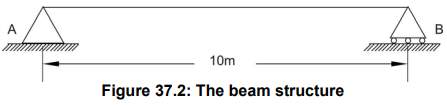Solution:
As discussed earlier, there are two ways this problem can be solved. Both the approaches will be demonstrated here.

Tabulate values:
As shown in the figure, a unit load is places at distance x from support A and the reaction value RB is calculated by taking moment with reference to support A. Let us say, if the load is placed at 2.5 m. from support A then the reaction RB can be calculated as follows (Figure 37.3).

Σ MA = 0 : RB x 10 - 1 x 2.5 = 0 ⇒ RB = 0.25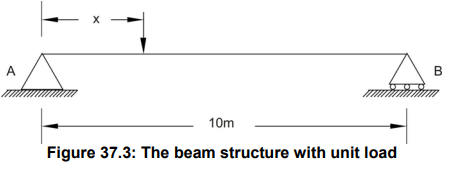Similarly, the load can be placed at 5.0, 7.5 and 10 m. away from support A and reaction RB can be computed and tabulated as given below.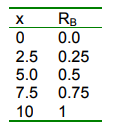Graphical representation of influence line for RB is shown in Figure 37.4.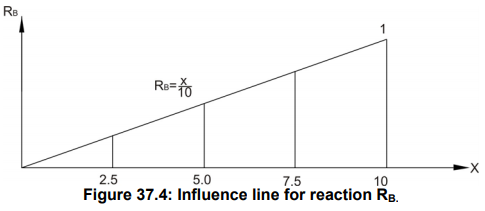Influence Line Equation:
When the unit load is placed at any location between two supports from support A at distance x then the equation for reaction RB can be written as

Σ MA = 0 : RB x 10 – x = 0 ⇒ RB = x/10

The influence line using this equation is shown in Figure 37.4.

Example 2:
Construct the influence line for support reaction at B for the given beam as shown in Fig 37.5.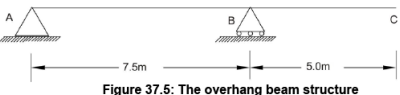Solution:
As explained earlier in example 1, here we will use tabulated values and influence line equation approach.

Tabulate Values:
As shown in the figure, a unit load is places at distance x from support A and the reaction value RB is calculated by taking moment with reference to support A. Let us say, if the load is placed at 2.5 m. from support A then the reaction RB can be calculated as follows.

Σ MA = 0 : RB x 7.5 - 1 x 2.5 = 0 ⇒ RB = 0.33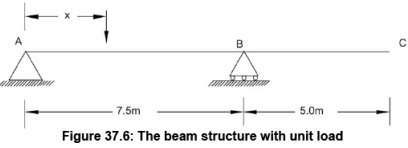Similarly one can place a unit load at distances 5.0 m and 7.5 m from support A and compute reaction at B. When the load is placed at 10.0 m from support A, then reaction at B can be computed using following equation.

Σ MA = 0 : RB x 7.5 - 1 x 10.0 = 0 ⇒ RB = 1.33

Similarly a unit load can be placed at 12.5 and the reaction at B can be computed. The values of reaction at B are tabulated as follows.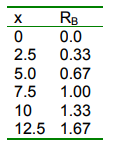Graphical representation of influence line for RB is shown in Figure 37.7.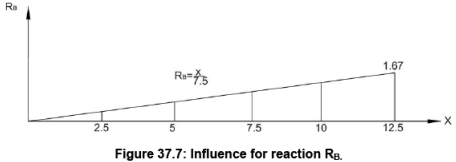Influence line Equation:

Applying the moment equation at A (Figure 37.6),

Σ MA = 0 : RB x 7.5 - 1 x x = 0 ⇒ RB = x/7.5

The influence line using this equation is shown in Figure 37.7.

Example 3:
Construct the influence line for shearing point C of the beam (Figure 37.8)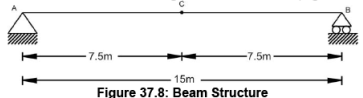Solution:
Tabulated Values:

As discussed earlier, place a unit load at different location at distance x from support A and find the reactions at A and finally computer shear force taking section at C. The shear force at C should be carefully computed when unit load is placed before point C (Figure 37.9) and after point C (Figure 37.10). The resultant values of shear force at C are tabulated as follows.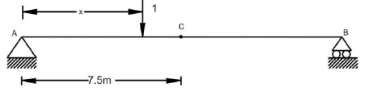Figure 37.9: The beam structure – a unit load before section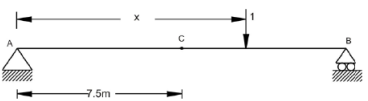Figure 37.10: The beam structure - a unit load before section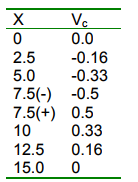Graphical representation of influence line for Vc is shown in Figure 37.11.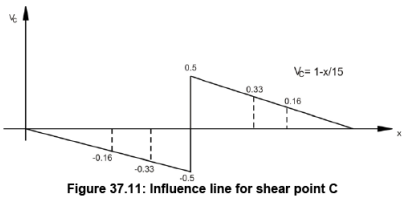Influence line equation:
In this case, we need to determine two equations as the unit load position before point C (Figure 37.12) and after point C (Figure 37.13) will show different shear force sign due to discontinuity. The equations are plotted in Figure 37.11.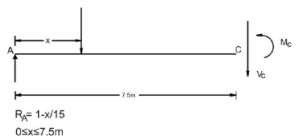Figure 37.12: Free body diagram – a unit load before section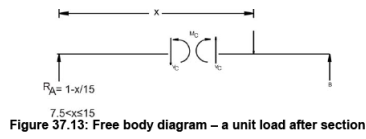Influence Line for Moment:
Like shear force, we can also construct influence line for moment.

Offer running on EduRev: Apply code STAYHOME200 to get INR 200 off on our premium plan EduRev Infinity!

## Structural Analysis

30 videos|122 docs|28 tests

,

,

,

,

,

,

,

,

,

,

,

,

,

,

,

,

,

,

,

,

,

;